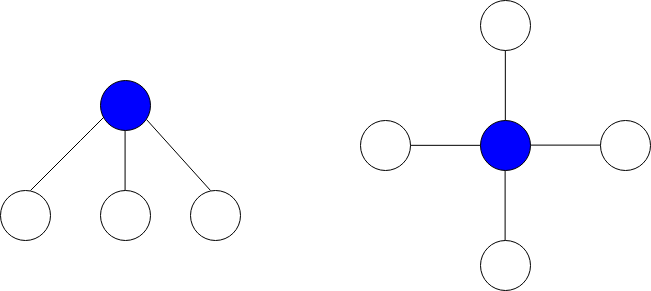##### Welcome to Subscribe On Youtube

Formatted question description: https://leetcode.ca/all/2497.html

# 2497. Maximum Star Sum of a Graph

• Difficulty: Medium.
• Related Topics: .
• Similar Questions: Number Of Ways To Reconstruct A Tree, Find Center of Star Graph.

## Problem

There is an undirected graph consisting of n nodes numbered from 0 to n - 1. You are given a 0-indexed integer array vals of length n where vals[i] denotes the value of the ith node.

You are also given a 2D integer array edges where edges[i] = [ai, bi] denotes that there exists an undirected edge connecting nodes ai and bi.

A star graph is a subgraph of the given graph having a center node containing 0 or more neighbors. In other words, it is a subset of edges of the given graph such that there exists a common node for all edges.

The image below shows star graphs with 3 and 4 neighbors respectively, centered at the blue node.The star sum is the sum of the values of all the nodes present in the star graph.

Given an integer k, return the **maximum star sum of a star graph containing at most k edges.**

Example 1:Input: vals = [1,2,3,4,10,-10,-20], edges = [[0,1],[1,2],[1,3],[3,4],[3,5],[3,6]], k = 2
Output: 16
Explanation: The above diagram represents the input graph.
The star graph with the maximum star sum is denoted by blue. It is centered at 3 and includes its neighbors 1 and 4.
It can be shown it is not possible to get a star graph with a sum greater than 16.


Example 2:

Input: vals = [-5], edges = [], k = 0
Output: -5
Explanation: There is only one possible star graph, which is node 0 itself.
Hence, we return -5.


Constraints:

• n == vals.length

• 1 <= n <= 105

• -104 <= vals[i] <= 104

• 0 <= edges.length <= min(n * (n - 1) / 2, 105)

• edges[i].length == 2

• 0 <= ai, bi <= n - 1

• ai != bi

• 0 <= k <= n - 1

## Solution (Java, C++, Python)

• class Solution {
public int maxStarSum(int[] vals, int[][] edges, int k) {
int n = vals.length;
List<Integer>[] g = new List[n];
Arrays.setAll(g, key -> new ArrayList<>());
for (var e : edges) {
int a = e, b = e;
if (vals[b] > 0) {
}
if (vals[a] > 0) {
}
}
for (var e : g) {
Collections.sort(e, (a, b) -> b - a);
}
int ans = Integer.MIN_VALUE;
for (int i = 0; i < n; ++i) {
int v = vals[i];
for (int j = 0; j < Math.min(g[i].size(), k); ++j) {
v += g[i].get(j);
}
ans = Math.max(ans, v);
}
return ans;
}
}

• class Solution {
public:
int maxStarSum(vector<int>& vals, vector<vector<int>>& edges, int k) {
int n = vals.size();
vector<vector<int>> g(n);
for (auto& e : edges) {
int a = e, b = e;
if (vals[b] > 0) g[a].emplace_back(vals[b]);
if (vals[a] > 0) g[b].emplace_back(vals[a]);
}
for (auto& e : g) sort(e.rbegin(), e.rend());
int ans = INT_MIN;
for (int i = 0; i < n; ++i) {
int v = vals[i];
for (int j = 0; j < min((int) g[i].size(), k); ++j) v += g[i][j];
ans = max(ans, v);
}
return ans;
}
};

• class Solution:
def maxStarSum(self, vals: List[int], edges: List[List[int]], k: int) -> int:
g = defaultdict(list)
for a, b in edges:
if vals[b] > 0:
g[a].append(vals[b])
if vals[a] > 0:
g[b].append(vals[a])
for bs in g.values():
bs.sort(reverse=True)
return max(v + sum(g[i][:k]) for i, v in enumerate(vals))


• func maxStarSum(vals []int, edges [][]int, k int) (ans int) {
n := len(vals)
g := make([][]int, n)
for _, e := range edges {
a, b := e, e
if vals[b] > 0 {
g[a] = append(g[a], vals[b])
}
if vals[a] > 0 {
g[b] = append(g[b], vals[a])
}
}
for _, e := range g {
sort.Sort(sort.Reverse(sort.IntSlice(e)))
}
ans = math.MinInt32
for i, v := range vals {
for j := 0; j < min(len(g[i]), k); j++ {
v += g[i][j]
}
ans = max(ans, v)
}
return
}

func max(a, b int) int {
if a > b {
return a
}
return b
}

func min(a, b int) int {
if a < b {
return a
}
return b
}


Explain:

nope.

Complexity:

• Time complexity : O(N * log N).
• Space complexity : O(N).